# Atomic-scale capacitance¶

Version: P-2019.03

In this tutorial you will perform an atomic-scale simulation of a parallel plate nano-capacitor, which means that the gap between the two parallel metal surfaces is in the nanometer range. This is currently not a realistic device, but the purpose is to show a methodology that can be used to compute the capacitance of other structures, including metal/semiconductor/metal structures.Attention

Capacitor physics
Applying a bias $$V$$ across the capacitor leads to a build-up of oppositely signed charge $$Q$$ on the plates, and therefore an electric field $$E$$ between them. The electric field strength can be written as $$E=\sigma/\varepsilon = V/d$$, where $$\sigma = Q/A$$ is the charge density and $$\varepsilon = k \varepsilon_0$$ is the permittivity of the spacer layer between the plates (vacuum or dielectric). The capacitance can then be calculated from $$C = Q/V = Q / Ed = A \varepsilon/d$$, where $$d$$ is the distance between the plates.

Calculations
The equations given above are valid for an ideal macroscopic parallel plate capacitor, but a nano-scale capacitor may in general be quite different. The main method used for computing the capacitance from first principles is to evaluate the electrostatic energy, and from that the capacitance. We will also show a slightly simpler approach based on the Mulliken charge populations of the atoms.## Build the parallel plate capacitor¶

Open the Builder, and do the following:

• Use Add ‣ From Database to add gold to the Stash.
• Open the Builders ‣ Cleave plugin, and create a Au(111) electrode by entering the Miller indices as .
• Repeat the structure 8 times in the C direction using Bulk Tools ‣ Repeat.
• Select and delete the two atoms in the middle – they have atom indices 19 and 4.
• Select the surface atoms (indices 10 and 11 after deletion), and turn them into ghost atoms using the toolbar icon.
• Turn the bulk structure into a device configuration via Device Tools ‣ Device From Bulk and use the default settings.

The result will be the device as shown below.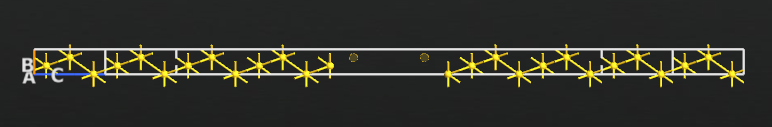Note

The ghost atoms are used to improve the description of the electron density between the two surfaces. They are simply extra basis sets placed in the empty region. This technique, which is sometimes referred to as a vacuum basis, is also used for calculating the work function of a surface, as explained in a tutorial: Computing the work function of a metal surface using ghost atoms.

## Calculations¶

### Zero bias¶

Send the device configuration to the Script Generator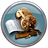by clicking the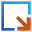icon, and set up the script as follows:

Send the script to the Job Manager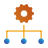and execute the calculation. It takes 3-4 minutes on a laptop.

### Finite bias¶

To compute the capacitance it is necessary to compute $$dq/dV$$, where $$dq$$ is the induced charge on the surfaces and $$V$$ the bias voltage. In the bias voltage you will also run the following analysis objects: MullikenPopulation, ElectrostaticDifferencePotential, and ElectronDifferenceDensity.

Use the following script to perform the calculations for bias voltages of 0.25, 0.5, 0.75, and 1.0 V:

device_configuration = nlread('au_vacuumgap.hdf5', DeviceConfiguration)[-1]
calculator = device_configuration.calculator()
for voltage in [0.0, 0.25, 0.5, 0.75, 1.0]:
# Set new calculator with modified electrode voltages on the configuration
# use the self consistent state of  the old calculation as starting input.
device_configuration.setCalculator(
calculator(electrode_voltages=(0.5*voltage*Volt, -0.5*voltage*Volt)),
initial_state=device_configuration)
device_configuration.update()
nlsave('au_vacuumgap.hdf5', device_configuration, object_id="SCF %s" % voltage)
# -------------------------------------------------------------
# Mulliken population
# -------------------------------------------------------------
mulliken_population = MullikenPopulation(device_configuration)
nlsave('au_vacuumgap.hdf5', mulliken_population, object_id="Mulliken %s" % voltage)
# -------------------------------------------------------------
# Electrostatic difference potential
# -------------------------------------------------------------
electrostatic_difference_potential = ElectrostaticDifferencePotential(device_configuration)
nlsave('au_vacuumgap.hdf5', electrostatic_difference_potential, object_id="EDP %s" % voltage)
# -------------------------------------------------------------
# Electron difference density
# -------------------------------------------------------------
electron_difference_density = ElectronDifferenceDensity(device_configuration)
nlsave('au_vacuumgap.hdf5', electron_difference_density, object_id="EDD %s" % voltage)


You can download the scipt here: bias_loop.py. Run it using the Job Manager or execute it from a terminal:

\$ atkpython bias_loop.py > bias_loop.out


It takes about half an hour to finish this calculation on a laptop.

Note

The previously converged calculation is used as an initial guess for the next calculation to speed up the SCF convergence. The object_id makes it easier to identify the data in post-SCF analysis.

## Analysis¶

We will introduce two different ways to calculate the capacitance:

• a simple approach based on the induced charge;
• a more general method based on evaluating the electrostatic energy.

### Approach based on the induced charge¶

The finite bias will build up a net positive/negative charge on the two gold surfaces, as shown in the plots below.Now, the capacitance can in principle be computed as $$C=dq/dV$$, where $$q$$ is the total accumulated charge on one surface as a function of the bias. From the slope of the straight line you can therefore obtain the capacitance of the nano-capacitor:The plots above, as well as the entire data analysis based on the Mulliken populations, can be achieved by running this script: mulliken-analysis.py. It is a long script but a great opportunity to learn about custom data analysis in Python and QuantumATK.

Results

The script reports a capacitance of 7.27*10-22 Farad, as computed from fitting a straight line to the data points. To put this number into perspective, consider the capacitance of an ideal parallel plate capacitor, written as $$C = \varepsilon A/d$$, where $$\varepsilon=1$$ for the vacuum permittivity and the area $$A$$ of each plate can be calculated from the device structure. You can then calculate the parallel plate distance $$d=A/C$$. This is also done in the script and it reports $$d$$=8.77 Å.

Comparing this value with the distance between the surface gold atoms (11.89 Å) the position of the so-called image plane is estimated to be 1.56 Å above the gold surface.

### Electrostatic energy analysis¶

To compute the capacitance using a more general and accurate method, you will need to first calculate the electrostatic energy $$E$$ as a function of the applied bias $$V$$:

$E(V)=\frac{1}{2}\int \delta v(\mathbf{r},V) \delta n(\mathbf{r}, V)d\mathbf{r}$

where $$\delta v$$ is the induced electrostatic potential and $$\delta n$$ is the induced density.

The script potential_plot.py extracts the potential and density at 1 V bias from the existing HDF5 file and produces the plots of the induced density and electrostatic potential. The script also reports two parameters:

• the electric field in the middle of the vacuum gap $$E$$= 0.1127 V/Å;
• the distance between the two image planes $$d$$=8.88 Å.

Tip

The position of the image planes is defined as the point where the electric field is zero, that is, where the electrostatic potential becomes flat.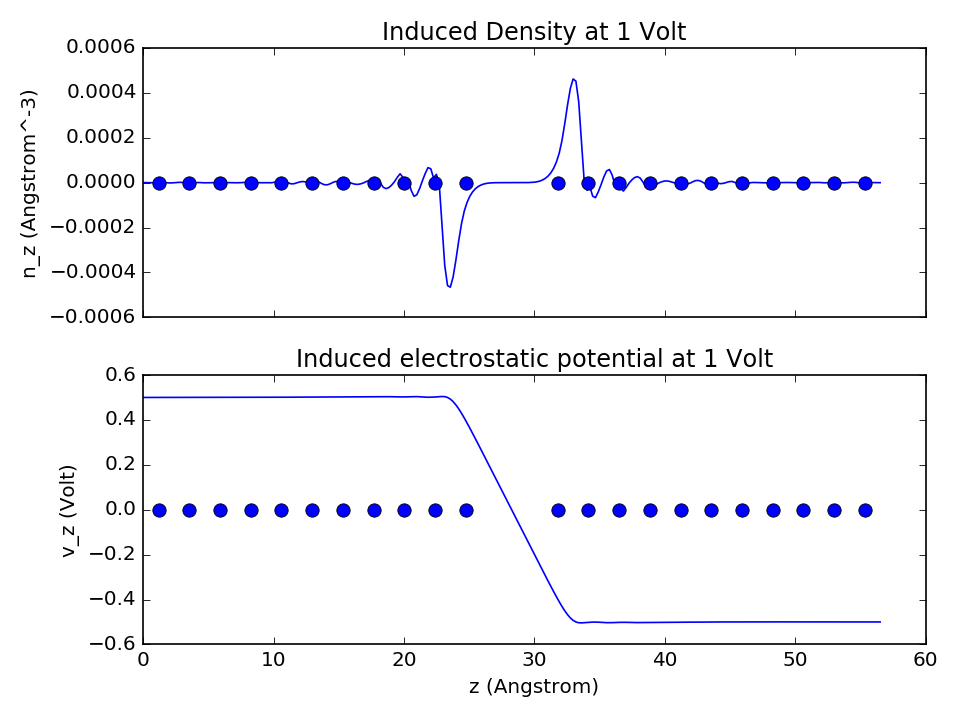The blue circles correspond to the atom positions. The main charge accumulation takes place in a very narrow region right above the surface, between the outer surface atoms and the ghost atoms.

From this, the capacitance can be extracted by fitting a parabola to the relation $$E(V) = CV^2/2$$. The script v-e-plot.py calculates the electrostatic energy and fits the capacitance as shown below.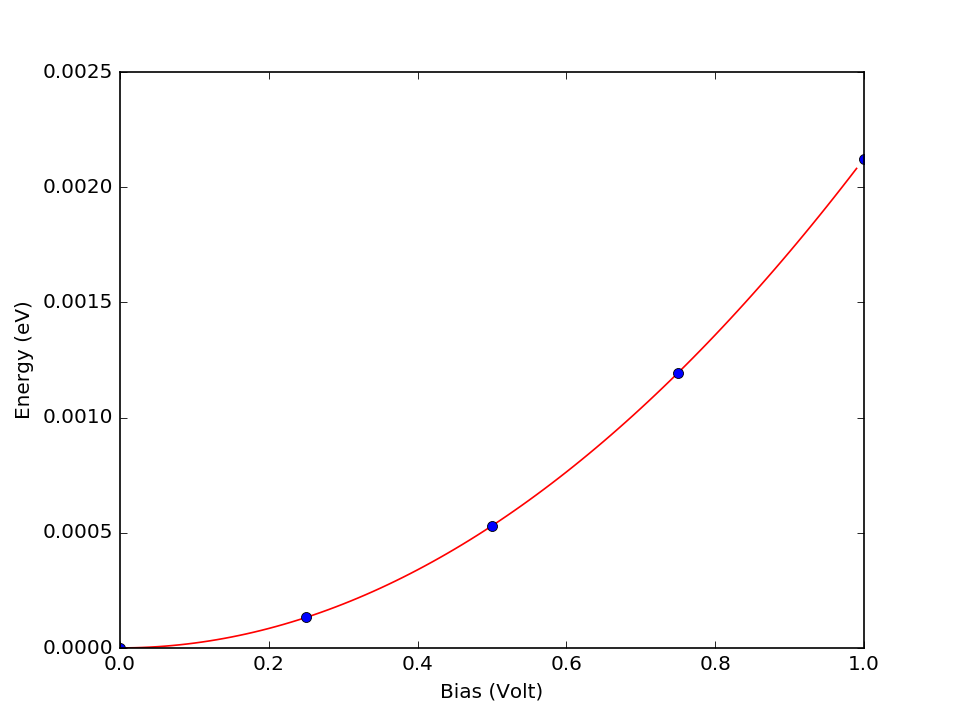Results

The script reports the capacitance $$C$$=6.81*10-22 Farad, in qualitative agreement with the value obtained from the simple approach using Mulliken charge analysis.

This script also reports $$d$$=9.36 Å, corresponding to an image plane at 1.27 Å above the gold surface, in very close accordance with the position of the peak of induced density.

Attention

What is the difference between the two methods?
In the Mulliken charge analysis, it is assumed that all electrons on the left surface have the same potential, and similarly for the right surface. When we convolute the electron density and the electrostatic potential we account for the fact that some part of the electron density is located in the region with a finite electric field. The electrostatic energy analysis is therefore more accurate for calculating the capacitance.

The electrostatic energy analysis also has the advantage that it does not require the researcher to separate the atoms as uniquely “left” and “right” – this is trivial for the two gold surfaces, but that may not always be the case.

## Bias-dependent capacitance¶

The analysis outlined above is based on the assumption that the capacitance is independent of the voltage. However, this is not always the case. A more advanced spline curve fitting allows you to investigate systems whose capacitance does depend on the bias voltage.

Provided that you have already run the scripts mulliken-analysis.py and v-e-plot.py in the previous section, you can now download and run the script v-c-plot.py to perform the fitting.

Note

The data saved by the two scripts are used by the present script to accelerate the analysis.

The computed capacitance is shown below as a function of the bias. As expected, the capacitance changes in this case very little with the bias voltage.Attention

The present example uses rather few data points to illustrate the method. In general, you should use more than five data points to get an accurate analysis.

## Dielectric spacer material¶

The electric field in the vacuum gap is extremely high (over 900*106 V/m at 1 V bias) and would immediately lead to a breakdown discharge in the device. Replacing the vacuum gap between the capacitor plates with a dielectric material can partly solve this.

There are two different ways to do this using QuantumATK:

1. Insert a dielectric material, and explicitly form the two metal–semiconductor–metal interfaces:
• Spatial region: Use the QuantumATK Builder to place a dielectric spatial region between the surfaces.
2. Use a cheaper and less rigorous approach, where you simply modify the dielectric constant of the region between the gold surfaces:
• Implicit solvent method: Assign a dielectric constant different than 1 to the vacuum region by modifying settings in the Poisson solver.

For demonstration purposes, we here go for the first option.

Note

In both cases it is necessary to use the Multigrid Poisson solver, but you can still have periodic boundary conditions along A and B.

### Dielectric spatial region¶

Follow the steps that led to calculation of the capacitance from electrostatic energy analysis, but add the dielectric spatial region and change the Poisson solver to Multigrid.

To use a dielectric spatial region, go to the Builderand use the Miscellaneous ‣ Spatial Regions tool to place between the surfaces a box with a relative dielectric constant of 10: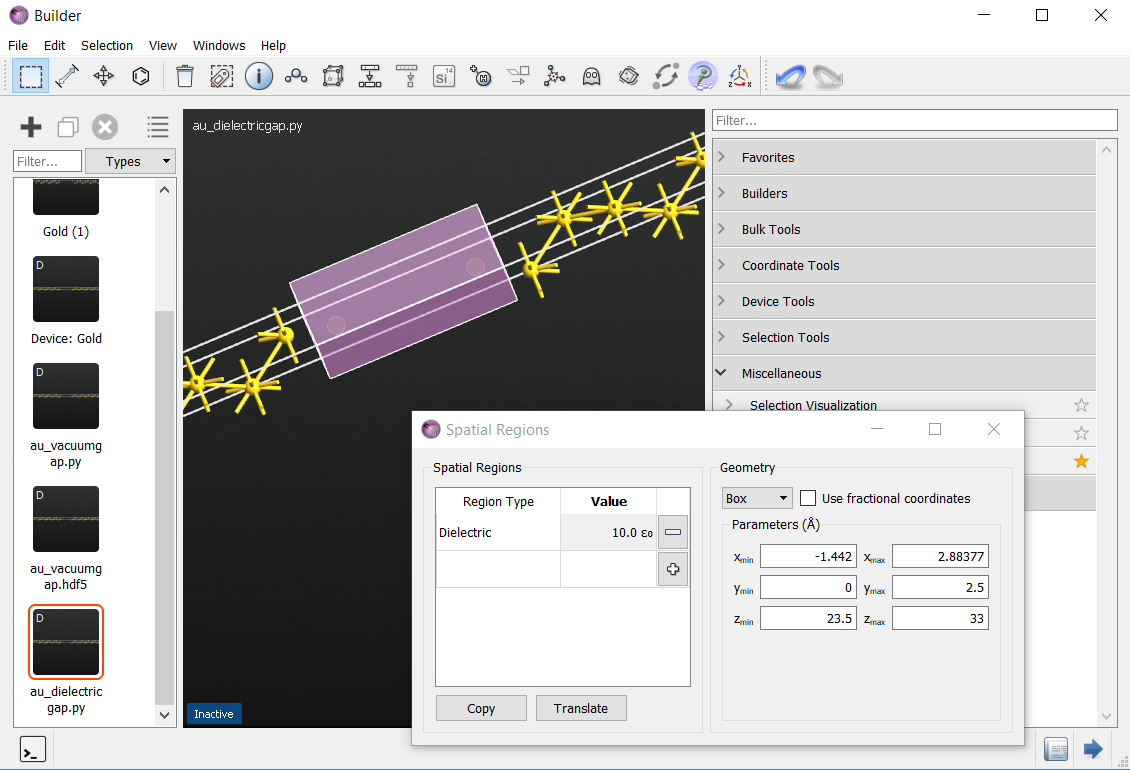Note

The dielectric region is a rectangular box, while the unit cell is hexagonal for the present  fcc surface. To solve the mismatch, make sure the rectangular box is large enough in the AB plane to extend outside the unit cell. The parts outside the cell will be cut off during the calculation.

Then send the new geometry to the Script Generatorand repeat all the steps. Or simply open the structure in the Editor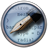and copy/paste the relevant parts of the scripts you already have, and change the names of the output files.

The results are illustrated below. The position of the dielectric region is indicated by the grey shading. You can clearly see the expected discontinuity of the electric field near the edges of the dielectric region, and you will find that the capacitance has significantly increased.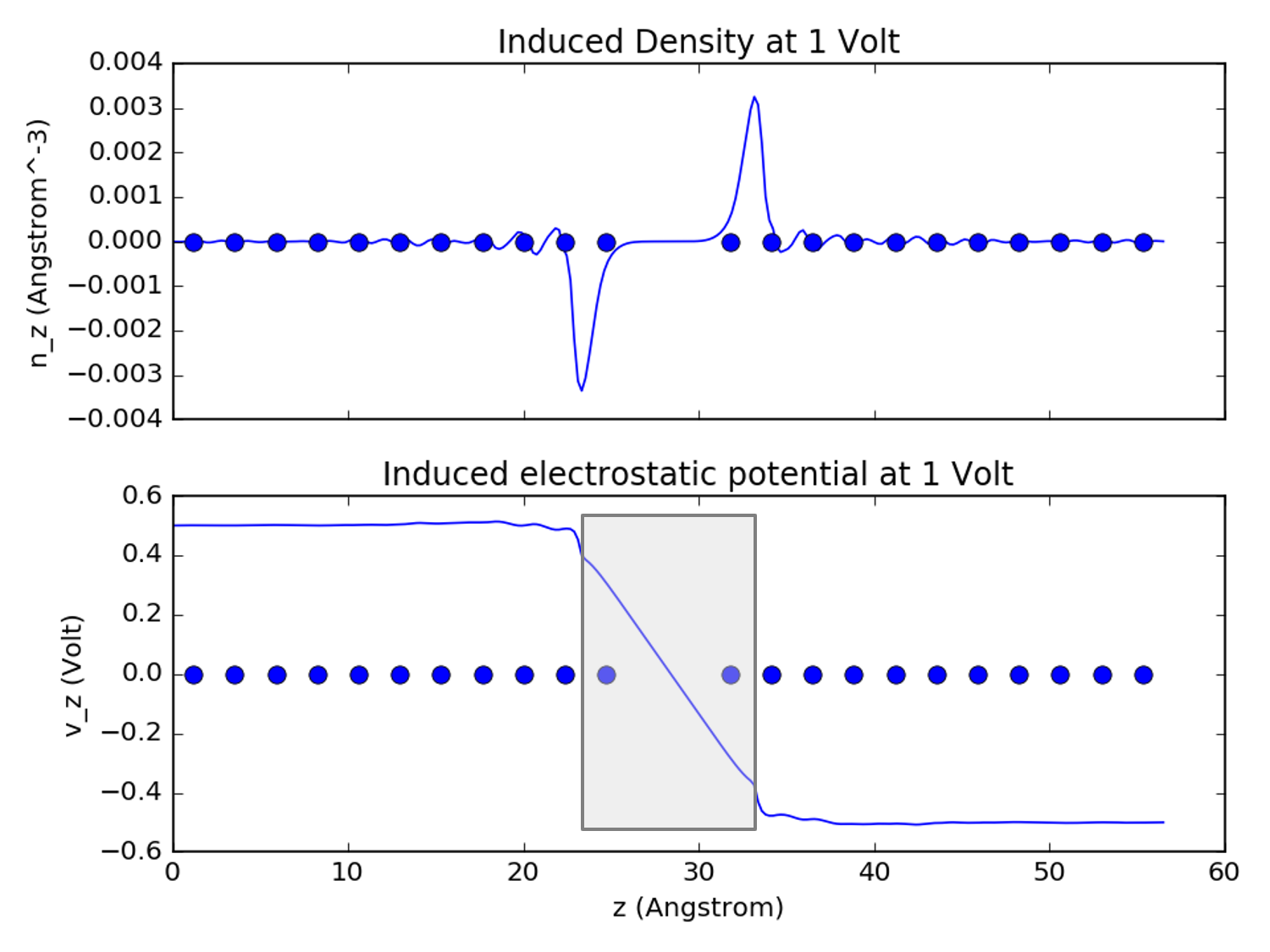Note

• The scripts used in the electrostatic energy analysis are self-contained and generic. They can be used to analyse other systems as well, after suitable modification of the file names.
• The scripts used in the Mulliken charge analysis need to be modified before they can be used for other systems, to account for geometry changes.
• These approaches are valid only if the current through the device is very small. For example, the transmission is extremely small (it’s a capacitor – not a diode!). You can verify that the transmission is extremely small for this large vacuum gap, of the order 10-23.

Tip

What next?
Another interesting example of a nano-scale capacitor would be a one formed by Carbon Nanotube Junctions.